Name:    2nd Grade Operations and Algebraic Thinking- Work with equal groups of objects to gain foundations for multiplication, Standard 3, Pre Test 1 Determine whether a group of objects (up to 20) has an odd or even number of members, e.g., by pairing objects or counting them by 2s; write an equation to express an even number as a sum of two equal addends.

 CompletionComplete each statement. 1. Count the objects by 2”s and give the answer below:  @% @% @% @% 2. Count the objects by 2’s and give the answer below:  ! # © Ÿ \$ W € É < * 1 Ç Ü 3. Count the objects by 2’s and give the answer below:  F            FF   FFF          F                           F               F        F 4. Count the objects by 2’s and give the answer below:  X                                X     X                  XX                                           X                               X            X   X   X                                            X 5. Is the number of items below and odd or even amount?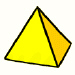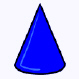6. Is the number of items below and odd or even amount?                                                            j£¢€€¢¢j     ¢        j         €                         ££€£                        j                              j   €                                            € 7. Are there an odd or even number of school items below?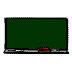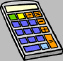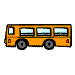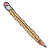8. Is the sum of these two numbers odd or even?7 + 6 ? 9. Is the sum of these two numbers odd or even?9 + 9 ? 10. Is the sum of these two numbers odd or even?6  + 6 ? 11. Is the sum of these two numbers odd or even?3 + 8 ? 12. Which of the following statements is most true? (Put only the letter of the statement in the answer space.)a)  The sum of two addends (numbers)  is always even.b)  The sum of two addends that are equal is always even.c)  The sum of two addends that are not equal is always odd.d)  An even number added to an uneven number is always even.